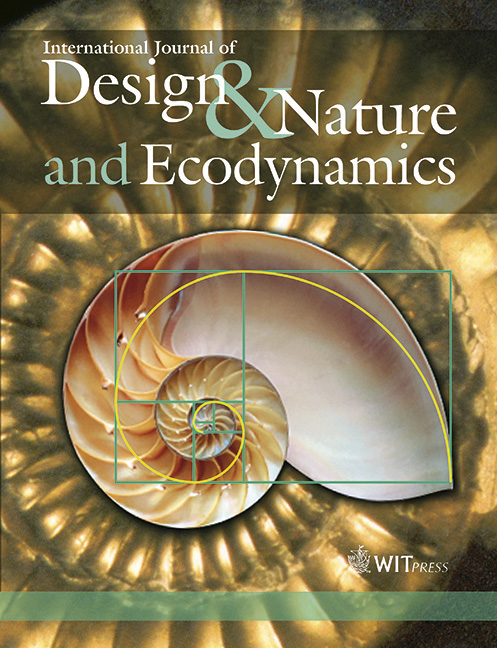WIT Press

MATHEMATICAL ASPECTS OF THE MECHANICS OF LEFT VENTRICULAR CONTRACTION

Price

Free (open access)Volume

Volume 5 (2010), Issue 2

Pages

15

Page Range

173 - 188

Paper DOI

10.2495/DNE-V5-N2-173-188

WIT Press

Author(s)

R.M. SHOUCRI

Abstract

Large elastic deformation is used in order to model the mechanics of left ventricular contraction. The active force generated by the myocardium is modeled as force/unit myocardial volume in the mathematical formalism describing the local equilibrium of forces in the myocardium. Expressions for the stress components are derived by assuming a cylindrical geometry for the left ventricle, the total stress is expressed as the sum of a component due to the deformation of the passive medium of the myocardium and an active component induced by the tension in the muscular fibers. It is shown that knowledge of the tension generated by the muscular fiber in the myocardium can lead to useful information for the estimation of the pseudo-strain energy function used to express the stress-strain constitutive relations in a non-linear model.

Keywords

active force of the myocardium, cardiac mechanics, mathematical modeling of ventricular contraction, pressure-volume relation in the left ventricle